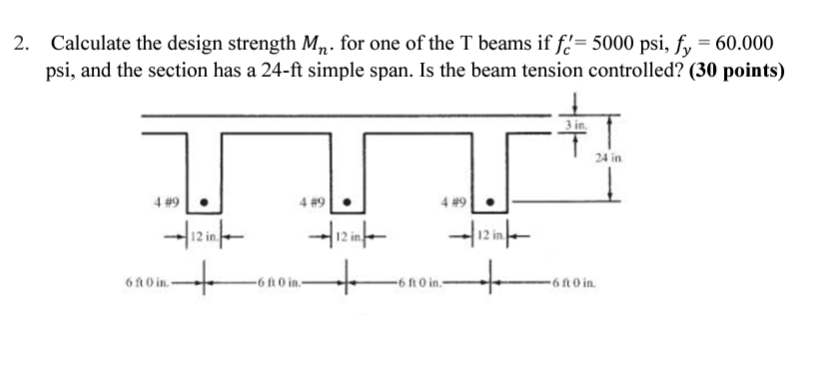Home / Expert Answers / Civil Engineering / 2-calculate-the-design-strength-mn-for-one-of-the-t-beams-if-fc-5000psi-fy-60-000-pa802

# (Solved): 2. Calculate the design strength Mn. for one of the T beams if fc=5000psi,fy=60.000 ...2. Calculate the design strength . for one of the T beams if psi, and the section has a simple span. Is the beam tension controlled? (30 points)

We have an Answer from Expert